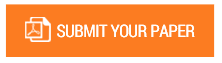Simulation of the Cardiovascular Mechanical System Based on Pressure-Flow Model Rest Condition

Authors : Dimas Primasatya; Erry Rimawan; Hendi Herlambang; Horas Canman S

Volume/Issue : Volume 5 - 2020, Issue 7 - July

Scribd : https://bit.ly/3jf3YTg

DOI : 10.38124/IJISRT20JUL031

Non-invasive measurement method has made rapid developments in the field of biomedical engineering. One of research is impedance cardiography (ICG), which provide information of pulsation basic. By knowing this kind of measurement technique, it will assist inspection of the patient's physiological condition with cardiovascular system. This research is aimed to determine the mechanical characteristics of the cardiovascular system in the human body such as a wave graph of pressure, flow, and volume, based on pressure–flow model in rest condition, and analyze the simulation results by implementing state of the physiology cardiovascular disease. To obtain the wave chart that is modeled by the cardiovascular system using a lumped parameter method, formulate the differential equations of the pressure–flow dynamics equation for an incompressible fluid in a segment of a cylindrical elastic tube and simulate the model using the Simulink toolboxes from Matlab R2008b. The simulation with lumped parameter method resulted wave graphics of pressure, flow, and volume of physiological state a person in rest condition, the left ventricular pressure is 120 mmHg , right ventricular pressure is 30 mmHg , left ventricular outflow is 800 mL / sec and volume in the left ventricle is 160 mL . By implementing the simulation have been developed on the physiological state of cardiovascular disease, hypertension occurs when the arteries resistance R3i = 0.61 mmHg × s mL with the pressure of the left ventricle is 145 mmHg. For coronary heart condition, ventricular pressure decreased until 82 mmHg in the value of the coronary arteries resistance is R3o = 0.852 mmHg × s mL. This research assumed heart haves the character of passive because there is no feedback signal that can compensate if the pressure in the systemic circulation is reduced. The research can be concluded that the graph from simulation shows the results are not much different from the reference chart, this results indicates that the equation and the simulation was able to reflect on the human circulatory physiological circumstances. A little different of a graphic simulation result due to differences in the parameters and assumptions used.

Keywords : Cardiovascular Mechanical Systems, Simulation, Pressure, Flow

#### CALL FOR PAPERS

Paper Submission Last Date
31 - December - 2023